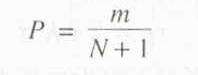Categories

# FLOOD

A flood is an unusually high stage in a river normally the level at which the river overflows its banks and inundates the adjoining area.

The damages caused by floods  in  terms  of  loss  of  life,  property  and  economic  loss  due  to  disruption  of economic activity are all too well known.

Crores of rupees are spent every year in flood control and flood forecasting.

The hydrograph of extreme floods and stages corresponding to flood peaks provide valuable data for purposes of hydrologic design.

Further, of the various characteristics of the flood hydrograph, probably the most important and widely used parameter is the flood peak.

At a given location in a stream, flood peaks vary from year to year and their magnitude constitutes a hydrologic series which enable one to assign  a  frequency  to  a  given  flood-peak  value.

In  the  design  of  practically  all hydraulic structures the peak flow that can be expected with an assigned frequency (say 1 in 100 years) is of primary importance to adequately proportion the structure to accommodate its effect.

The design of bridges, culvert waterways and spillways for dams and estimation of scour at a hydraulic  structure are some examples  wherein flood-peak values are required.

To estimate the magnitude of a flood peak the following alternative methods are available:

Rational method, empirical method, unit-hydrograph, and flood-frequency studies.

The use of a particular method depends upon (i) the desired objective, (ii) the available data and (iii) the importance of the project.

Further the rational formula is only applicable to small site (< 50 m) catchments and the unit-hydrograph method is normally restricted to moderate size catchments with areas less than 5000 km.

## FLOOD FREQUENCY STUDIES

Hydrologic processes such as floods are exceedingly complex natural events.

They  are resultants  of a number  of component  parameters  and are  therefore  very difficult, analytically.

For example, the buds in a catchment depend upon the characteristics of the catchment, rainfall and antecedent conditions, each one of these factors in turn depend upon a host of constituent parameters.

This makes the elimination of the flood peak a very complex problem leading to many different approaches.

The empirical formulae and unit-hydrograph methods presented  in  the  previous  sections  are  some  of  them.  Another  approach  to  the prediction of flood flows, and also applicable to other hydrologic processes such as rainfall etc. is the statistical method of frequency analysis.The values  of the maximum  flood  from a given  catchments  area for large number    of successive  years  constitute  a  hydrologic  data  series  called  the annual series.

The  data  are  then  arranged  in  decreasing  order  of  magnitude  and  the probability  P  of  each  event  being  equaled  to  or  exceeded  (plotting  position)  is calculated by the plotting position formula

Where M = order number of the event and

N = total number of events in the data.

The recurrence  interval, T(also called the return period or frequency) is calculated as T=1/P

The last column shows the return period 1 of various flood magnitude, Q. A plot of Q vs T yields the probability distribution.

For small return periods (i.e. for interpolation) or where limited extrapolation is required, a simple best fitting curve through plotted points can be used as the probability distribution.

A logarithmic scale for T is often advantageous. However, when larger extrapolations of Tare  involved,  theoretical  probability  distributions  have  to  be  used.

In  frequency analysis of floods the usual problem is to predict extreme flood events. Towards this, specific  extreme-value  distributions  are assumed  and the required  statistical parameters calculated from the available data.

Using these  the flood magnitude for a specific return period is estimated.

Chow  (1951)  has shown that most  frequency  distribution functions applicable to hydrologic studies can be expressed by the following equation known as the general  equation of hydrologic frequency analysis:

where   x  = value of the variate X of a random hydrologic series with a return period T

x  = mean of the variate

= standard deviation of the variate

K = frequency  factor which depends upon the return period, T

and the assumed frequency  distribution.

Some of the commonly used frequency distribution function  predication of extreme flood values are

• Gumbel’ s extreme —v alue distribution
• Log —Pearson Type II distribution
• Log normal distribution